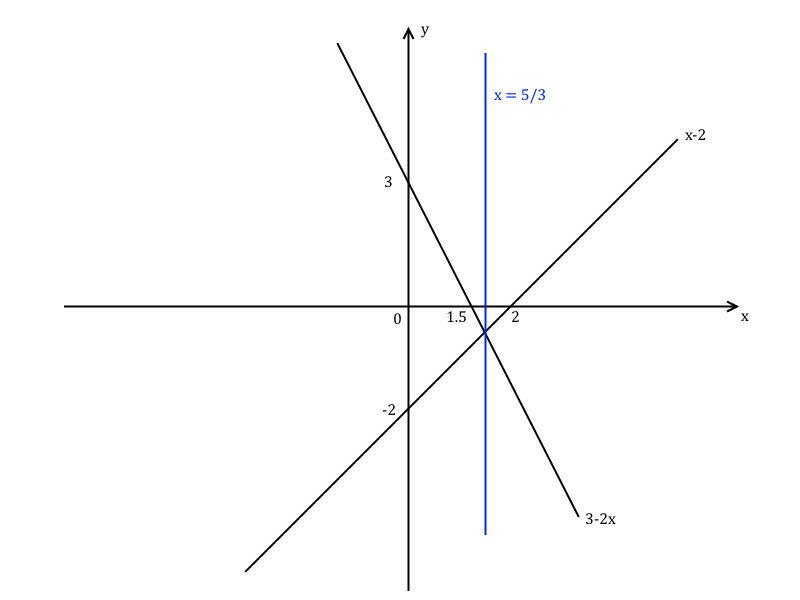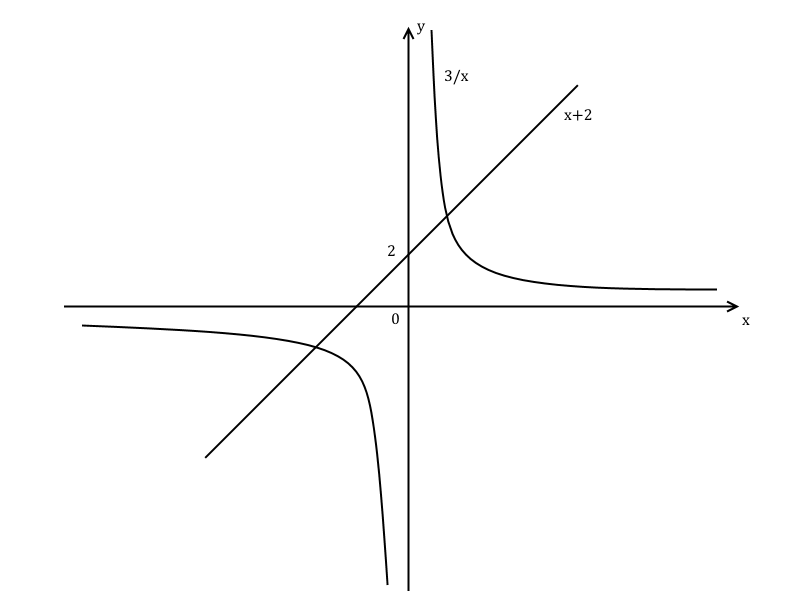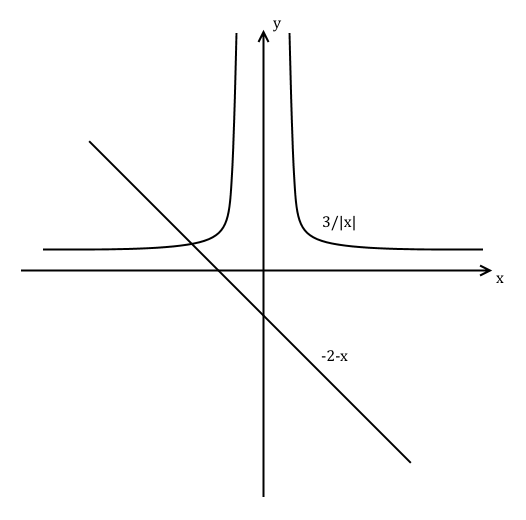# Inequalities

## Introduction

In FP2 you will be asked to solve inequalities. Contrary to solving an equation by getting a single value, the solution to an inequality is usually a range of values instead.

You will be given two expressions, one greater than the other, and you will need to find the range of values for which it is true. By this point in your A-level maths studies you will no doubt be familiar with the inequality symbols $\gt$, $\ge$, $\lt$, $\le$.

You can solve inequalities analytically using simple algebraic manipulation. Get all the $x$ terms on one side and solve for $x$. But you will also be asked to solve them graphically. This is actually somewhat more descriptive of what an inequality is geometrically, i.e. finding the set of points for which one expression is bigger than the other.

There are also some things you can't do with inequalities that you can when solving an equation. If you multiply or divide both sides by a negative number, you must reverse the inequality. This is logical - you are flipping the signs of both sides.

Also, raising the expressions to a power is problematic. The square of a number $|x| \lt 1$ is always less than the original number, e.g. $0.5^{2}=0.25\lt 0.5$. But the square of a number $|x| \gt 1$ is always greater than the original number, e.g. $10^{2} = 100 \gt 10$. The same problem exists for nth roots.

### Example

Q) Solve $x-2 \gt 3-2x$ graphically.

A) First we need to see if the lines represented by $x-2$ and $3-2x$ meet.

\begin{align} x-2 &= 3-2x \\ 2x + x &= 3 + 2 \\ 3x &= 5 \\ \therefore x &= \frac{5}{3} \end{align}

So the two lines meet at $x = \frac{5}{3}$. Then draw the graph of the two lines. $x-2$ meets the $y$-axis at $y=-2$ and the $x$-axis at $x=2$. $3-2x$ meets the $y$-axis at $y=3$ and the $x$-axis when $3-2x=0 \Rightarrow x = \frac{3}{2}$.And you can see from the graph that $x-2$ is bigger than $3-2x$ for $x \gt \frac{5}{3}$. So we've solved the inequality.

### Example

Q) Solve the above example again analytically.

A) Simply move the $x$ terms to one side and solve

\begin{align} x-2 & \gt 3-2x \\ 3x & \gt 5 \\ x & \gt \frac{5}{3} \end{align}

And that's the same answer we came to before. The analytical way is sometimes much faster but the graphical method can be more descriptive and explanatory.

### Example

Q) Solve $x+2\ge \frac{3}{x}$ graphically.

A) Let's start by drawing the lines represented by $x+2$ and $\frac{3}{x}$.You can see that the $x+2$ line is bigger than the $\frac{3}{x}$ over two segments of $x$. The first segment is between the point where the lines first meet and the $y$-axis, and the second segment is from the second point where they meet again on to infinity.

So let's find the intersection points of $x+2$ and $\frac{3}{x}$.

\begin{align} x+2 &= \frac{3}{x} \\ x^{2}+2x &= 3 \\ x^{2}+2x-3 &= 0 \\ \left(x+3\right)\left(x-1\right) &= 0 \end{align}

Solving this yields $x=-3, 1$. So $x+2\ge \frac{3}{x}$ when $-3 \le x \lt 0$ and $x \ge 1$.

### Example

Q) Solve the above example again analytically.

A) Move everything to one side so we get a quadratic expression. Unlike the previous graphical example where we used an equality to check where the two lines met, we don't simply multiply both sides by $x$, because there are $x \lt 0$.

\begin{align} x+2 & \ge \frac{3}{x} \\ x+2 - \frac{3}{x} & \ge 0 \\ \frac{x\left(x+2\right)-3}{x} & \ge 0 \\ \frac{x^{2}+2x-3}{x} & \ge 0 \\ \frac{\left(x+3\right)\left(x-1\right)}{x} & \ge 0 \end{align}

So we need to find values of $x$ such that $\frac{\left(x+3\right)\left(x-1\right)}{x} \ge 0$. We see that the critical values of the left hand expression are $x=-3$, $x=0$, and $x=1$. We will test certain values of $x$ in the expression $\frac{\left(x+3\right)\left(x-1\right)}{x}$ and see if it results in a positive or negative value.

Right off the bat, we can immediately see that the values $x=-3, 1$ satisfy the 'equals' part of the $\ge$ inequality. But we'll try values that are just above/below the three critical values.

$x=-3.1$:

$$\frac{\left(-\right)\left(-\right)}{\left(-\right)} \lt 0$$

$x=-2.9$:

$$\frac{\left(+\right)\left(-\right)}{\left(-\right)} \gt 0$$

$x=-0.1$:

$$\frac{\left(+\right)\left(-\right)}{\left(-\right)} \gt 0$$

$x=0.1$:

$$\frac{\left(+\right)\left(-\right)}{\left(+\right)} \lt 0$$

$x=0.9$:

$$\frac{\left(+\right)\left(-\right)}{\left(+\right)} \lt 0$$

$x=1.1$:

$$\frac{\left(+\right)\left(+\right)}{\left(+\right)} \gt 0$$

So from that we can see that $x+2\ge \frac{3}{x}$ for $-3 \le x \lt 0$ and $x \ge 1$.

## Modulus inequalities

In FP2 you also need to solve inequalities where either or both of the expressions contain a modulus sign. You must then consider the cases when the terms inside the modulus signs are positive and negative.

Recall that when drawing a graph with modulus signs, the graph of $|f\left(x\right)|$ is the graph of $f\left(x\right)$ with the negative values of $f\left(x\right)$ reflected across the $x$-axis, and that the graph of $f\left(|x|\right)$ is the graph of $f\left(x\right)$ with all values of $f\left(x\right)$ for $x \gt 0$ reflected across the $y$-axis, i.e. $f\left(-x\right)=f\left(x\right)$. Also recall that if $k$ is a constant, $k|x|=|kx|$.

### Example

Q) Solve $\frac{3}{|x|} \gt -2 - x$ graphically.

A) Firstly draw the graphs of $\frac{3}{|x|}$ and $-2 - x$. Note that $\frac{3}{|x|} = \left|\frac{3}{x}\right|$.Clearly $\frac{3}{|x|}$ is bigger than $-2 - x$ to the right of the point where the two lines meet, somewhere in the negative $x$-axis.

So let's find where they meet. First take the case where $x \gt 0$.

\begin{align} \frac{3}{x} &= -2-x \\ 3 &= -2x -x^{2} \\ x^{2} + 2x + 3 &= 0 \end{align}

This won't help us since the quadratic has no real roots. This makes sense since the graph shows that the lines only meet where $x\lt 0$. So then take the case where $x \lt 0$.

\begin{align} \frac{3}{-x} &= -2-x \\ 3 &= 2x +x^{2} \\ x^{2} + 2x - 3 &= 0 \\ \left(x+3\right)\left(x-1\right) &= 0 \end{align}

So we have $x=-3$ and $x=1$ which is absurd since we took $x \lt 0$. The place where the two lines meet is therefore at $x=-3$. Therefore $\frac{3}{|x|} \gt -2 - x$ for $x \gt -3$.